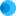Intuit HelpIntuit

# Accumulated depreciation shows in Investing Activities on the EasyACCT cash flow statement

SOLVEDby IntuitEasyACCTUpdated October 06, 2021

EasyACCT checks the current year activity in the Accumulated Depreciation account and matches it to the Depreciation Expense account. Any differences between these accounts will be printed in the Investing Activities section.

## Follow these steps to troubleshoot the problem:

1. Make sure the amount of Depreciation Expense and the change in Accumulated Depreciation are the same.
• Change in Accumulated Depreciation is calculated by taking the balance at the end of the prior year, minus the balance at the end of the current year.
• If these accounts differ, then Accumulated Depreciation will appear in the investing section on the Statement of Cash Flows.
2. Review the chart of accounts structure.
• All fixed asset accounts should come first, then all accumulated depreciation accounts.
• The Cash Flow will be incorrect if the chart of accounts is set up with a fixed asset account, then a corresponding accumulated depreciation account.
• The recommended setup is described in EasyACCT help on setting up the chart of accounts.
3. Make sure the Accumulated Depreciation and Depreciation Expense account ranges are correct in the financial statement setup.
• If using Statement Templates:
1. Go to Write-Up, Update Company Files, Financial Statements, and then Statement Template.
2. Select on the Cash Flow tab.
3. Make sure the beginning and ending account numbers are correct for  Accumulated Depreciation and Depreciation Expense.
• If using Custom Statements:
1. Go to Write-Up, Update Company Files, Financial Statements, and then Custom Statements.
2. Locate the instruction number just before the description "Accumulated Depreciation." This account range should be the Accumulated Depreciation accounts.
3. Locate the instruction line before the description "Accumulated Depreciation." This account range should be the Depreciation Expense account.
4. Make sure the Sold Asset lines on the Cash Flow tab are completed.
• If this isn't entered, EasyACCT doesn't know where to end the Sales (Purchases) of Assets section. Therefore, it will use the first Accumulated Depreciation account as the ending.
• If the Sold Assets accounts doesn't exist, change the first Accumulated Depreciation account to the one before the current account. For example, if the first Accumulated Depreciation account is 1700, change it to 1699. If 1699 is an account in use, it will need to be renumbered to a different account number.